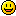Scripts / [SOLVED] Gate sideway (left or right)

Message
Posted: 28th Mar 2021 20:32
Hello,
i have found a script to move a wall vertical.
But is there a way to move it left or right ?
Here is the script i have found:
--------------------------------------------
local starty = {}
local move_speed = {}
local max_move = {}
local activate_range = {}
local close_range = {}
local moved = {}
local state = {}
local door_name = {}
local delay = {}

--name door y2 to move down (otherwise it defaults to up)
function door_vertical_init_name(e,name)
door_name[e] = name
state[e] = "closed"

--how fast the door slides up/down
move_speed[e] = 1
--how far the door will move in total
max_move[e] = 150
--how close the player gets before the door will open
activate_range[e] = 200
--how far away the player needs to be before the door will shut again
close_range[e] = 600
--if "animation" is still too quick adjust delay here
delay[e] = 1

end

function door_vertical_main(e)

if starty[e] == nil then
starty[e] = g_Entity[e]['y']
if door_name[e] == "y2" then
move_speed[e] = -move_speed[e]
--adjusts close range so that door works better when moving down
close_range[e] = close_range[e] * 4
end
else

if state[e] == "closed" then
if GetPlayerDistance(e) < activate_range[e] then
moved[e] = 0
StartTimer(e)
state[e] = "opening"
end

elseif state[e] == "opening" then
if GetTimer(e) > delay[e] then
StartTimer(e)
if moved[e] < max_move[e] then
moved[e] = moved[e] + 1
CollisionOff(e)
SetPosition(e,g_Entity[e]['x'],g_Entity[e]['y']+move_speed[e],g_Entity[e]['z'])
CollisionOn(e)
else
state[e] = "open"
end
end
if GetPlayerDistance(e) > close_range[e] then
state[e] = "closing"
end

elseif state[e] == "open" then
CollisionOff(e)
if GetPlayerDistance(e) > close_range[e] then
StartTimer(e)
state[e] = "closing"
end

elseif state[e] == "closing" then
if GetTimer(e) > delay[e] then
StartTimer(e)
if moved[e] > 0 then
moved[e] = moved[e] - 1
CollisionOff(e)
SetPosition(e,g_Entity[e]['x'],g_Entity[e]['y']-move_speed[e],g_Entity[e]['z'])
if moved[e] < max_move[e] * 0.8 then
CollisionOn(e)
end
else
state[e] = "closed"
end
end
if GetPlayerDistance(e) < activate_range[e] then
state[e] = "opening"
end

end --state

end --setup
end --main

The author of this post has marked a post as an answer.

Posted: 29th Mar 2021 11:38
This post has been marked by the post author as the answer.
This should work

Posted: 29th Mar 2021 15:48
Find this in the script:
SetPosition(e,g_Entity[e]['x'],g_Entity[e]['y']+move_speed[e],g_Entity[e]['z'])
'Y' is the vertical part going up ------------/\. . . . . . . /\
put "move_speed[e]" to a different tangent -------|

SetPosition( e, g_Entity[e]['x'], g_Entity[e]['y'], g_Entity[e]['z'] + move_speed[e] )
----------------------------set at 'Z' movement---------------------------------------/\

Default view in GG = "north" which is positive 'Z' tangent.
So reverse that is negative 'Z' tangent.
Left, right (west, east) = negative, positive 'X' respectively.
Posted: 29th Mar 2021 19:06
THX for help, this works great